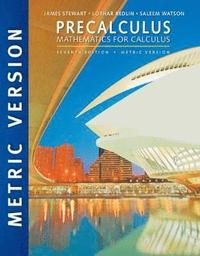Fler böcker inom
Format
Språk
Engelska
Antal sidor
1024
Utgivningsdatum
2016-01-01
Upplaga
7
Förlag
Brooks Cole
Medarbetare
Redlin, Lothar / Watson, Saleem
Dimensioner
217 x 276 x 35 mm
Vikt
2146 g
Antal komponenter
1
ISBN
9781305999985

### Du kanske gillar# Precalculus: Mathematics for Calculus, 7e, International Metric Edition

939
Skickas inom 3-6 vardagar.
Fri frakt inom Sverige för privatpersoner.
With its clear and simple writing style, PRECALCULUS: MATHEMATICS FOR CALCULUS, 7E, INTERNATIONAL METRIC EDITION, will give you a solid foundation in the principles of mathematical thinking. Problem solving and mathematical modeling are reinforced throughout. This comprehensive, evenly paced book provides complete coverage of the function concept and integrates substantial graphing calculator materials that help you develop insight into mathematical ideas. Online resources available with the text give you the practice you need to improve your grade in the course.

## Passar bra ihop

1.2. +
3.De som köpt den här boken har ofta också köpt College Algebra av James Stewart (inbunden).

## Kundrecensioner

Har du läst boken? Sätt ditt betyg »

## Övrig information

The late James Stewart received his M.S. from Stanford University and his Ph.D. from the University of Toronto. He did research at the University of London and was influenced by the famous mathematician George Polya at Stanford University. Stewart was most recently Professor of Mathematics at McMaster University, and his research field was harmonic analysis. Stewart was the author of a best-selling calculus textbook series published by Cengage Learning, including CALCULUS, CALCULUS: EARLY TRANSCENDENTALS, and CALCULUS: CONCEPTS AND CONTEXTS, as well as a series of precalculus texts. Lothar Redlin grew up on Vancouver Island, received a Bachelor of Science degree from the University of Victoria, and a Ph.D. from McMaster University in 1978. He subsequently did research and taught at the University of Washington, the University of Waterloo, and California State University, Long Beach. He is currently Professor of Mathematics at The Pennsylvania State University, Abington Campus. His research field is topology. Saleem Watson received his Bachelor of Science degree from Andrews University in Michigan. He did graduate studies at Dalhousie University and McMaster University, where he received his Ph.D. in 1978. He subsequently did research at the Mathematics Institute of the University of Warsaw in Poland. He also taught at The Pennsylvania State University. He is currently Professor of Mathematics at California State University, Long Beach. His research field is functional analysis.

## Innehållsförteckning

Preface.
To the Student.
Prologue: Principles of Problem Solving.
1. FUNDAMENTALS.
Chapter Overview.
Real Numbers. Exponents and Radicals. Algebraic Expressions. Rational Expressions. Equations. Complex Numbers. Modeling with Equations. Inequalities. The Coordinate Plane; Graphs of Equations; Circles. Lines. Solving Equations and Inequalities Graphically. Modeling Variation. Chapter 1 Review. Chapter 1 Test.
FOCUS ON MODELING: FITTING LINES TO DATA.
2. FUNCTIONS.
Chapter Overview. Functions. Graphs of Functions. Getting Information from the Graph of a Function. Average Rate of Change of a Function. Linear Functions and Models. Transformations of Functions. Combining Functions. One-to-One Functions and Their Inverses. Chapter 2 Review. Chapter 2 Test.
FOCUS ON MODELING: MODELING WITH FUNCTIONS.
3. POLYNOMIAL AND RATIONAL FUNCTIONS.
Chapter Overview. Quadratic Functions and Models. Polynomial Functions and Their Graphs. Dividing Polynomials. Real Zeros of Polynomials. Complex Zeros and the Fundamental Theorem of Algebra. Rational Functions. Polynomial and Rational Inequalities. Chapter 3 Review. Chapter 3 Test.
FOCUS ON MODELING: FITTING POLYNOMIAL CURVES TO DATA.
4. EXPONENTIAL AND LOGARITHMIC FUNCTIONS.
Chapter Overview. Exponential Functions. The Natural Exponential Function. Logarithmic Functions. Laws of Logarithms. Exponential and Logarithmic Equations. Modeling with Exponential Functions. Logarithmic Scales. Chapter 4 Review. Chapter 4 Test. FOCUS ON MODELING: FITTING EXPONENTIAL AND POWER CURVES TO DATA.
5. TRIGONOMETRIC FUNCTIONS: UNIT CIRCLE APPROACH.
Chapter Overview. The Unit Circle. Trigonometric Functions of Real Numbers. Trigonometric Graphs. More Trigonometric Graphs. Inverse Trigonometric Functions and Their Graphs. Modeling Harmonic Motion. Chapter 5 Review. Chapter 5 Test.
FOCUS ON MODELING: FITTING SINUSOIDAL CURVES TO DATA.
6. TRIGONOMETRIC FUNCTIONS: RIGHT TRIANGLE APPROACH.
Chapter Overview. Angle Measure. Trigonometry of Right Triangles. Trigonometric Functions of Angles. Inverse Trigonometric Functions and Triangles. The Law of Sines. The Law of Cosines. Chapter 6 Review. Chapter 6 Test.
FOCUS ON MODELING: SURVEYING.
7. ANALYTIC TRIGONOMETRY.
Chapter Overview. Trigonometric Identities. Addition and Subtraction Formulas. Double-Angle, Half-Angle, and Sum-Product Formulas. Basic Trigonometric Equations. More Trigonometric Equations. Chapter 7 Review. Chapter 7 Test.
FOCUS ON MODELING: TRAVELING AND STANDING WAVES.
8. POLAR COORDINATES AND PARAMETRIC EQUATIONS.
Chapter Overview. Polar Coordinates. Graphs of Polar Equations. Polar Form of Complex Numbers; DeMoivres Theorem. Plane Curves and Parametric Equations. Chapter 8 Review. Chapter 8 Test.
FOCUS ON MODELING: The Path of a Projectile.
9. VECTORS IN TWO AND THREE DIMENSIONS .
Chapter Overview. Vectors in Two Dimensions. The Dot Product. Three Dimensional Coordinate Geometry. Vectors in Three Dimensions. The Cross Product. Equations of Lines and Planes. Chapter 9 ...